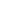Share

# What are kilobytes, megabytes, and gigabytes?

Answer: The size of computer data is measured in bytes. Just like a kilometer is a measure of 1000 meters, kilobytes, megabytes, and gigabytes are larger measurements of bytes. These units use SI prefixes, which is short for the "International System of Units." The correlation of kilobytes, megabytes, and gigabytes is listed below:

1. kilobyte = 1000 (or 1024) bytes
2. megabyte = 1000 (or 1024) kilobytes
3. gigabyte = 1000 (or 1024) megabytes
If a gigabyte is 1024 megabytes and a kilobyte is 1024 bytes, a gigabyte is 1,073,741,824 bytes. This is slightly larger than 1,000,000,000 bytes, which is often used as an estimate for a gigabyte. For an explanation of why these units are sometimes multiplied by 1024 and not 1000, view this Help Center article.

To avoid this ambiguity, data can also be expressed in Kibibytes, Mebibytes, and Gibibytes, which are always multiplied by 1024. For more information, view this Help Center article.

In case you're wondering, the units continue to go up in multiples of 1000 after gigabyte. Here is the complete list:

1. byte (B)
2. kilobyte (KB) - 1000^1
3. megabyte (MB) - 1000^2
4. gigabyte (GB) - 1000^3
5. terabyte (TB) - 1000^4
6. petabyte (PB) - 1000^5
7. exabyte  (EB) - 1000^6
8. zettabyte (ZB) - 1000^7
9. yottabyte (YB) - 1000^8
A yottabyte is a lotta bytes!

#### Published: March 4, 2005 — by Per Christensson

Answer from the PC Help Center
https://pc.net/helpcenter/kilobytes_megabytes_and_gigabytes